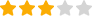Multiple Choice MultiplicationMultiple Choice Multiplication
• Statistics:
• players have already played this game!
• In brief:
• A colorful game in which you can practice multiplication tables in three different ways. The menu gives you three options: there is a black question mark in a different location in each game, and you have to replace the question marks with the right number to make the equations complete. These are multiple choice questions where you are shown four answers, with only one of these being correct. For each right answer you will receive one point and a new equation to solve. You have about 5 seconds per question.
• Enter a rating:
•(10)